# Force DiagramsPage 1

#### WATCH ALL SLIDES

Slide 1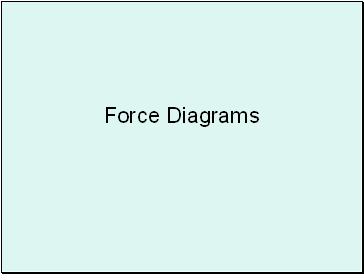Force Diagrams

Slide 2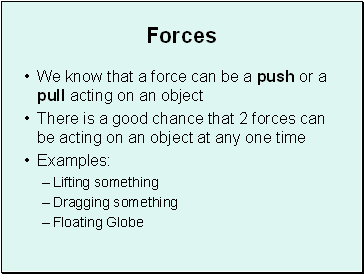## Forces

We know that a force can be a push or a pull acting on an object

There is a good chance that 2 forces can be acting on an object at any one time

Examples:

Lifting something

Dragging something

Floating Globe

Slide 3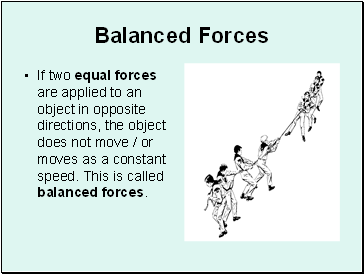## Balanced Forces

If two equal forces are applied to an object in opposite directions, the object does not move / or moves as a constant speed. This is called balanced forces.

Slide 4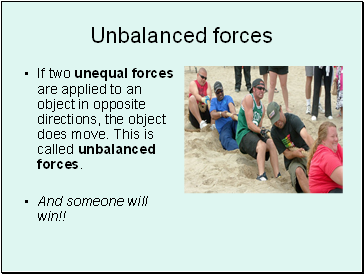## Unbalanced forces

If two unequal forces are applied to an object in opposite directions, the object does move. This is called unbalanced forces.

And someone will win!!

Slide 5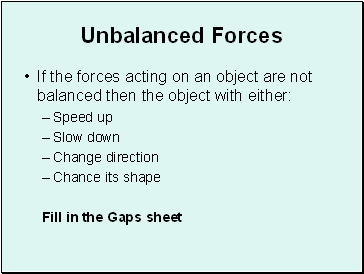Unbalanced Forces

If the forces acting on an object are not balanced then the object with either:

Speed up

Slow down

Change direction

Chance its shape

Fill in the Gaps sheet

Slide 6We can use force diagrams to show this:

4N

7N

The block has 2 opposing forces being applied to it:

7N to the right and 4N to the left. They are Unbalanced Forces.

To Calculate the Resultant Force (Fr),

subtract one from the other: 7N – 4N = 3N to the right

3N

Slide 7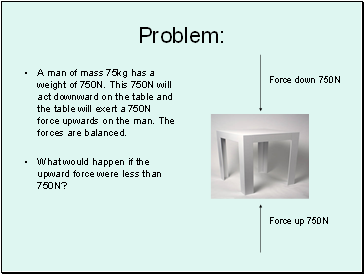## Problem

A man of mass 75kg has a weight of 750N. This 750N will act downward on the table and the table will exert a 750N force upwards on the man. The forces are balanced.

What would happen if the upward force were less than 750N?

Force down 750N

Force up 750N

Slide 8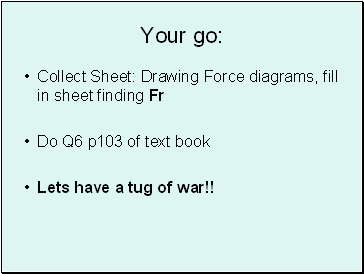Collect Sheet: Drawing Force diagrams, fill in sheet finding Fr

Do Q6 p103 of text book

Lets have a tug of war!!

Slide 9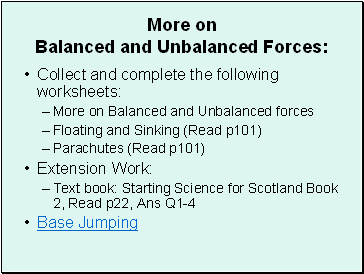More on Balanced and Unbalanced Forces:

Collect and complete the following worksheets:

More on Balanced and Unbalanced forces